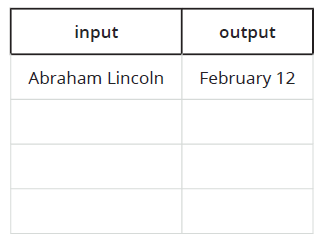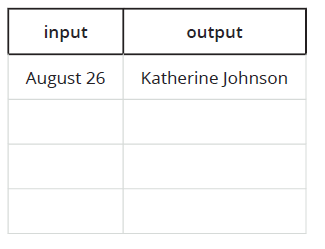# A.4.2.3 Birthdays

Rule B takes a person’s name as its input, and gives their birthday as the output. Complete the table by listing three more examples of input-output pairs. You can list them like this (Abraham Lincoln, February 12)If you use your name as the input to B, how many outputs are possible? Explain how you know.

Rule P takes a date as its input and gives a person with that birthday as the output. Complete the table by listing three more examples of input-output pairs. You can list them like this (August 26, Katherine Johnson)If you use your birthday as the input to , how many outputs are possible? Explain how you know.

Only one of the two relationships is a function. The other is not a function. Which one is which? Explain how you know.

For the relationship that is a function, write two input-output pairs from the table using function notation.